# The Earth

The Earth's surface is 510,000,000 km2. Calculates the radius, equator length, and volume of the Earth, assuming the Earth has the shape of a sphere.

r =  6370.5973 km
o =  40027.6436 km
V =  1083001548067.1 km3

### Step-by-step explanation: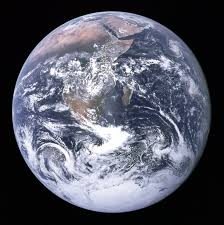Did you find an error or inaccuracy? Feel free to write us. Thank you!Tips to related online calculators
Tip: Our volume units converter will help you with the conversion of volume units.

#### You need to know the following knowledge to solve this word math problem:

We encourage you to watch this tutorial video on this math problem:

## Related math problems and questions:

• Oceans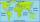The Earth's surface is approximately 510,000,000 km2 and is 7/10 covered by oceans. Of which 1/2 covers the Pacific Ocean, the Atlantic Ocean 1/4, the Indian Ocean 1/5 and the Arctic Ocean 1/20. What parts of the Earth's surface cover each ocean?
• Earth rotation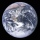How fast is the place on the Earth's equator moving if the Earth's radius is 6378 km?
• Earth's diameter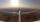The Earth's diameter on the equator is approximately 12750 km. How long does the Gripen fly over the Earth above the equator at 10 km if it is at an average speed of 1500 km / h?
• Volume of sphereHow many times does the volume of a sphere increase if its radius increases 2 ×?How many times does the surface of a sphere decrease if we reduce its radius twice?
• Earth parallelEarth's radius is 6370 km long. Calculate the length parallel of latitude 50°.
• Equator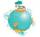Suppose that tourist went on foot over the Globe equator. How many meters more track made ​​his hat on his head as the shoes on your feet? The radius of the earth is 6378 km, and the height of the tourist is 1.7 m.Determine the radius of the circle if its perimeter and area is the same number.
• AirplaneAviator sees part of the earth's surface with an area of 200,000 square kilometers. How high he flies?
• Two hemispheres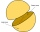In a wooden hemisphere with a radius r = 1, a hemispherical depression with a radius r/2 was created so that the bases of both hemispheres lie in the same plane. What is the surface of the created body (including the surface of the depression)?
• Elevation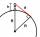What must be the elevation of an observer in order that he may be able to see an object on the earth 536 km away? Assume the earth to be a smooth sphere with radius 6378.1 km.
• Area to volumeIf the surface area of a cube is 486, find its volume.
• Pentagonal prismThe regular pentagonal prism is 10 cm high. The radius of the circle of the described base is 8 cm. Calculate the volume and surface area of the prism.
• TerezaThe cube has an area of base 256 mm2. Calculate the edge length, volume, and area of its surface.
• SurveyorsSurveyors mark 4 points on the surface of the globe so that their distances are the same. What is their distance from each other?
• Perimeter of the circleCalculate the perimeter of the circle in dm, whose radius equals the side of the square containing 0.49 dm2?
• Earth's surface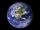The greater part of the earth's surface (r = 6371 km) is covered by oceans; their area is approximately 71% of the Earth's surface. What is the approximate area of the land?